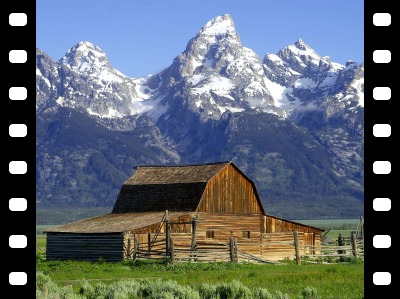## PERFORATIONS

 Applies film-like perforations to two opposite edges of an image.

 USAGE: perforations [-n number] [-s size] [-p position] [-b border] infile outfile USAGE: perforations [-h or -help] -n ... number ..... number of perforations; integer>0; default=8 -s ... size ....... size of perforations as percentage of smaller image ................... dimension; 00. The default=8. -s size ... SIZE of perforations as percentage of smaller image dimension. Values are 0

### EXAMPLES

 Example 1 -- Top/Bottom Edges (source) OriginalArguments: -n 8 -s 6 -p tb -b blackArguments: -n 8 -s 6 -p tb -b agedArguments: -n 8 -s 6 -p tb -b fadedArguments: -n 8 -s 6 -p tb -b noneExample 2 -- Left/Right Edges (source) OriginalArguments: -n 8 -s 6 -p lr -b blackArguments: -n 8 -s 6 -p lr -b agedArguments: -n 8 -s 6 -p lr -b fadedArguments: -n 8 -s 6 -p lr -b noneWhat the script does is as follows for border=black at the top/bottom edges: Creates a white round rectangle in a black background and tiles it out to make a row/column of the size of an edge of the image Composites the tiled row/column at two opposite edges of the image This is equivalent to the following IM commands ww=`convert \$infile -ping -format "%w" info:` hh=`convert \$infile -ping -format "%h" info:` mind=`convert xc: -format "%[fx:min(\$ww,\$hh)]" info:` ht=`convert xc: -format "%[fx:round(\$mind*\$size/100)]" info:` wd=`convert xc: -format "%[fx:round(\$ratio*\$ht)]" info:` rd=`convert xc: -format "%[fx:round(\$rndfact*(\$wd+\$ht)/2)]" info:` wd1=\$((wd-1)) ht1=\$((ht-1)) cellw=`convert xc: -format "%[fx:floor(\$ww/\$number)]" info:` cellh=`convert xc: -format "%[fx:floor(2*\$ht)]" info:` tilew=`convert xc: -format "%[fx:\$number*\$cellw]" info:` gravity1="north" gravity2="south" midw=\$ww midh=\$((hh-2*cellh)) flipping="-flip" appending="-append" blurring="blur:0x\${cellh}+90" shaving="0x2" convert -size \${wd}x\${ht} xc:black -fill white \ -draw "roundrectangle 0,0 \$wd1,\$ht1 \$rd,\$rd" -alpha off \ -gravity center -background black -extent \${cellw}x\${cellh} \ -write mpr:cell +delete -size \${tilew}x\${cellh} tile:mpr:cell \ -extent \${ww}x\${cellh} \$tmpA2 convert \$tmpA1 \$tmpA2 \( +clone \$flipping \) \ \( -clone 0 -clone 1 -gravity \$gravity1 -compose over -composite \) \ -delete 0,1 +swap -gravity \$gravity2 -compose over -composite \$outfile